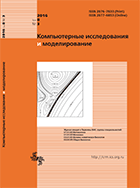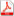Issue 3, 2016 Vol. 8

# All issues

Integration the relativistic wave equations in Bianchi IX cosmology modelpdf (135K)  / List of references

We consider integration Clein–Gordon and Dirac equations in Bianchi IX cosmology model. Using the noncommutative integration method we found the new exact solutions for Taub universe.

Noncommutative integration method for Bianchi IX model is based on the use of the special infinite-dimensional holomorphic representation of the rotation group, which is based on the nondegenerate orbit adjoint representation, and complex polarization of degenerate covector. The matrix elements of the representation of form a complete and orthogonal set and allow you to use the generalized Fourier transform. Casimir operator for rotation group under this transformation becomes constant. And the symmetry operators generated by the Killing vector fields in the linear differential operators of the first order from one dependent variable. Thus, the relativistic wave equation on the rotation group allow non-commutative reduction to ordinary differential equations. In contrast to the well-known method of separation of variables, noncommutative integration method takes into account the non-Abelian algebra of symmetry operators and provides solutions that carry information about the non-commutative symmetry of the task. Such solutions can be useful for measuring the vacuum quantum effects and the calculation of the Green’s functions by the splitting-point method.

The work for the Taub model compared the solutions obtained with the known, which are obtained by separation of variables. It is shown that the non-commutative solutions are expressed in terms of elementary functions, while the known solutions are defined by the Wigner function. And commutative reduced by the Klein–Gordon equation for Taub model coincides with the equation, reduced by separation of variables. A commutative reduced by the Dirac equation is equivalent to the reduced equation obtained by separation of variables.

Keywords: noncommutative integration, Bianchi IX
Citation in English: Breev A.I., Shapovalov A.V., Kozlov A.V. Integration the relativistic wave equations in Bianchi IX cosmology model // Computer Research and Modeling, 2016, vol. 8, no. 3, pp. 433-443
Citation in English: Breev A.I., Shapovalov A.V., Kozlov A.V. Integration the relativistic wave equations in Bianchi IX cosmology model // Computer Research and Modeling, 2016, vol. 8, no. 3, pp. 433-443
DOI: 10.20537/2076-7633-2016-8-3-433-443
Views (last year): 5.

Full-text version of the journal is also available on the web site of the scientific electronic library eLIBRARY.RU

The journal is included in the Russian Science Citation Index

The journal is included in the List of Russian peer-reviewed journals publishing the main research results of PhD and doctoral dissertations.

International Interdisciplinary Conference "Mathematics. Computing. Education"

The journal is included in the RSCI

Indexed in Scopus